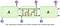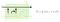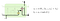# The Deep Learning Era — Part III

This the third post in the series of posts about my takeaways from understanding deep learning from a technical point-of-view.

Most machine learning data-sets have a sequential structure, such as in text documents certain words are more likely to appear in the data-set given a certain sequence of words preceding it. For example, the likelihood of the word “America” is increased if the previous words in sequence are “United States of”. Similarly online customers are more likely to buy a phone cover after buying a phone or a stock price is more likely to increase following a series of positive tweets about a company.

The objective of the problem decides how much sequential information is relevant. For example, in text classification, the sequential dependency is usually short range (one or two previous words) and thus 2-grams or 3-grams usually works well. Whereas for core natural language problems like Q&A, a matching answer to a question might depend on longer sequences of words forming the question.

For example, in an e-commerce conversational setting if a customer wants to know the price of 50" Samsung television, he/she can ask the question in multiple different ways.

1. What is the price of a 50" Samsung television ?
2. How much does a 50" Samsung television cost ?
3. How much do I need to pay in order to purchase a 50" Samsung television ?
4. Can you tell me the price of a 50" Samsung television ?
5. … and so on.

The goal of an intelligent agent would be to understand the query and map that query to appropriate historical queries in the chat database and then retrieve the best matching answer.

But there could be lot of similar questions, that is asking for a different answer, such as :

Can I get a 50" Samsung television below the price of \$1000 ?

Simple TF-IDF values of bag-of-words will work to some extent but cannot capture the “intent” of the question and thus will not be able to answer many questions correctly. The same problem will also be with a CNN architecture.

Although CNN works with word vectors, but word vectors capture “meaning” of individual words. Static CNN is constrained by the length of its sliding window. Our goal is to model sequence of words, i.e. generate a vector representation that captures the “meaning” of a sentence or a question or an answer and so on. Some possible solutions :

1. Concatenate word vectors for all words in a sequence. Maintains word order but not scalable to long sentences.
2. Paragraph Vectors or Doc2Vec. Inferred vector on test data not stable.
3. Dynamic Convolution Neural Networks.

Recurrent Neural Networks are the “deep learning alternatives” to sequence modeling algorithms such as HMM and Conditional Random Fields. These are like neural networks but inside a for-loop.

`words = nltk.word_tokenize(sentence)curr_out = numpy.zeros(64)for word in words:    curr_inp = U * curr_out + W * vector(word)    curr_out = NeuralNet(curr_inp)return curr_out`

Basically, it takes the word vector for the current word in the sentence and the hidden layer output from the previous word, computes their weighted sum and passes them through another hidden layer, to get the input for the next word and so on. You can think it of as a single neural network with number of hidden layers equal to the number of words in a sentence.

The final hidden layer output (from the last word) is considered to be the vector representation for the entire sentence.

The weights are updated using the back-propagation algorithm at the end of processing each sentence. Thus if a sentence is too long then the number of hidden layers would be large and the weights at the starting words would not be updated at all due to the vanishing gradient problem. LSTM comes to the rescue.Inside of an LSTM cell. Contains four different layers. (from colah.github.io)

So how does LSTM helps in considering very long range sequential dependencies while computing the final representation for a sentence ?

Think of a simple algorithmic problem :

You have an infinite stream of integers coming at you. Return the highest ‘K’ integer values at any point in time.

5 43 7 1 0 9 17 29 31 7 1 …..

To draw an analogy with our problem, assume that the highest ‘K’ integer values at any point in time is some kind of a representation for the entire stream at that point in time.

Obviously you cannot sort the integers every-time a new integer comes or cannot use a binary search tree to store all the integers seen, since the stream is infinite (or you can imagine that due to limited CPU memory, you cannot access the beginning of the stream)

So how do you keep a “memory” (or state) of what is relevant and what is irrelevant at any point in time with a limited amount of CPU ?

One possible solution is to use a min-heap of size K. After a new integer comes, it checks whether the heap is of size less than K. If the current size of heap is less than K, then by default insert this integer into heap, else if the heap size is already at K, then you have to decide whether the new integer should be among the top K or not.

Since the min-heap stores the smallest of the current highest K values in the root, compare the new integer with the root value and if the new integer is greater than the root value then it means that the new integer should be kept while discarding the root value. At any point in time the min-heap contains the highest K integer values seen so far.

`import heapqdef get_highest_k(new_num, min_heap, k):    if len(min_heap) < k:        heapq.heappush(min_heap, new_num)    else:        root = min_heap        if root < new_num:            heapq.heappop(min_heap)            heapq.heappush(min_heap, new_num)return min_heap`

LSTM essentially works on similar principle. Given a sequence of words, the hidden layer output for the current word is computed using only the latest “state” of the system. The latest “state” of the system is decided based on how much weightage to give to the previous state and how much weightage to a “possible” new state. Thus it is a “soft” update (unlike a “hard” update in the min-heap case, where the root value is either kept or discarded). One reason for “soft” update is that it is differentiable (requirement for back-propagation).New state Ct is an weighted average of previous state and possible new state.Current hidden layer output depends on the updated state of the cell.

But don’t read too much into the analogy as they solve completely different problems. The min-heap was used only for optimization purpose, the final output would be the same with or without the min-heap (assuming we had infinite amount of CPU), whereas in case of LSTM, the final output would be different due to some weights not being updated at all.

The difference between RNN and LSTM is that, whereas in RNN a single for loop contains a single sigmoid/tanh layer, LSTM contains fours different neural net layers. Also in LSTM apart from the hidden layer outputs at each word, we also have a sequence of “states” as seen above.

Written by